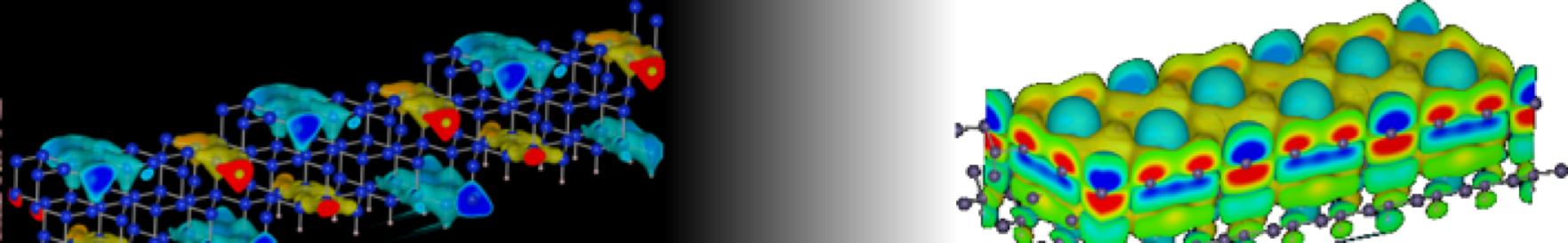SST Lab Dokuwiki Header#@surface

### サイト用ツール

jacapo:symmetry

set how symmetry is used to reduce k-points

:Parameters:

val : Boolean

 set_sym(True) Maximum symmetry is used
set_sym(False) No symmetry is used

This variable controls the if and how DACAPO should attempt using symmetry in the calculation. Imposing symmetry generally speeds up the calculation and reduces numerical noise to some extent. Symmetry should always be applied to the maximum extent, when ions are not moved. When relaxing ions, however, the symmetry of the equilibrium state may be lower than the initial state. Such an equilibrium state with lower symmetry is missed, if symmetry is imposed. Molecular dynamics-like algorithms for ionic propagation will generally not break the symmetry of the initial state, but some algorithms, like the BFGS may break the symmetry of the initial state. Recognized options:

“Off”: No symmetry will be imposed, apart from time inversion symmetry in recipical space. This is utilized to reduce the k-point sampling set for Brillouin zone integration and has no influence on the ionic forces/motion.

“Maximum”: DACAPO will look for symmetry in the supplied atomic structure and extract the highest possible symmetry group. During the calculation, DACAPO will impose the found spatial symmetry on ionic forces and electronic structure, i.e. the symmetry will be conserved during the calculation.

jacapo/symmetry.txt · 最終更新: 2022/08/23 13:34 by 127.0.0.1